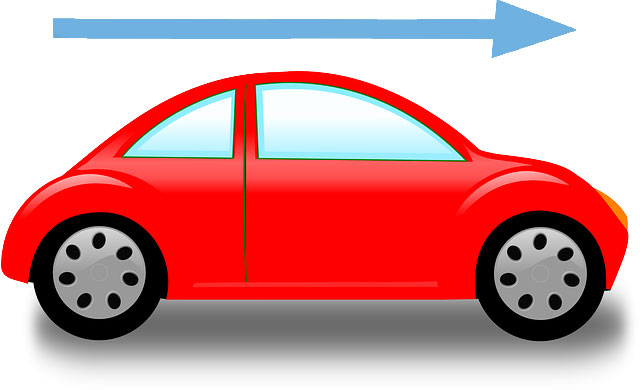## What is Speed?

Speed is the physical quantity for measuring the motion of a body with respect to a reference. It is defined as, the distance covered in a given amount of time.

For example, a car is traveling 50 km in an hour, then its speed would be 50 Km/h. Speed is a scalar quantity, means it doesn’t need a direction for its representation.

## What is Velocity?

Velocity is a little different than the speed. Velocity is a vector quantity, means it requires direction in which the body is moving along with the magnitude.

For example, a car is moving with a speed of 30 km/h in the north direction. Here you are given the direction (north) along with the magnitude which is 30 Km/h. You can say that the speed is the magnitude of the velocity.

## How are speed and velocity measured?

Velocity is measured in its standard unit which is meter per second (m/s). But, commonly we use Km/h (kilometer per hour) and mph (miles per hour). Velocity is calculated according to the following formula:

V = Δd/Δt = change in distance/change in time

or in other words

V = distance covered/amount of time

The magnitude of both speed and velocity is calculated in the same. But, velocity should also have a direction to properly define it.

Example: A car is moving towards west. It has covered 150 kilometers in 3 hours. Calculate its velocity.

Solution:

Distance covered = Δd = 150 km

Amount of time = Δt = 3 hours

Velocity = Δd/Δt = 150/3 = 50 km/h towards west

## Instantaneous velocity

When the velocity is measured at any particular time, it is called instantaneous velocity. A car’s speedometer always shows instantaneous velocity. Instantaneous velocity tells us the current speed of an object.

## Average Velocity

Average velocity is defined as, the total distance traveled by an object (e.g. a car) divided by the elapsed time.

For example, a car is traveling towards the north has covered a distance of 50 km in two hours. Then, its average velocity during the 50 km route will be V = 50/2 = 25 km/h. It doesn’t mean that the car was traveling all the time with the velocity of 50 km/h. Because there may be need of braking and accelerate the car, so the car may have traveled with different speed.

## Facts

• Light has the fastest possible speed. It’s speed is 299,792,458 meters/second or ‎186,000 miles/second.
• Escape velocity is the velocity, which when an object achieves can escape the gravitational pull of the planets.
• If you through an object towards the sky with a velocity of 11.2 kilometers/seconds, it will escape the gravitational pull of the Earth, and will never come back.
• When two objects with different weights are dropped from a height, both objects will hit the ground at the same time with same speed. It was proved by Galileo Galilei.